# 《黃昏之時，三葉的名字——由數學來守護》——2018數感盃 / 高中組專題報導類第三名

2018/04/13 | | 標籤：• 作者：楊子毅、吳冠宏／高雄市新莊高中

## 一、 研究緣起

「瀧、瀧」

──《你的名字》，小說，第 12 頁

「……本來想告訴妳……」
「不論妳在世界上的哪一個角落，我一定會再去見妳。」
──《你的名字》，小說，第 202 頁

## 三、探究分析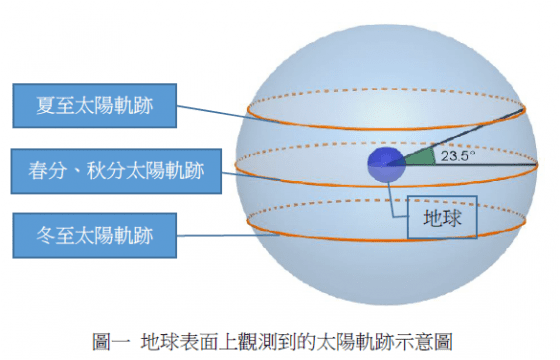$$\left\{\begin{matrix} x^2+y^2=cos^2\theta_{1}\\z=sin\theta_{1} \end{matrix}\right.$$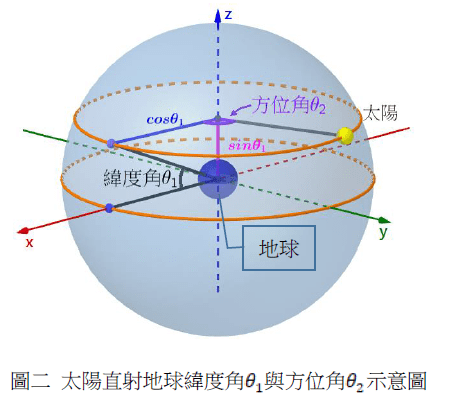(cosθ1cosθ2，cosθ1sinθ2，sinθ1)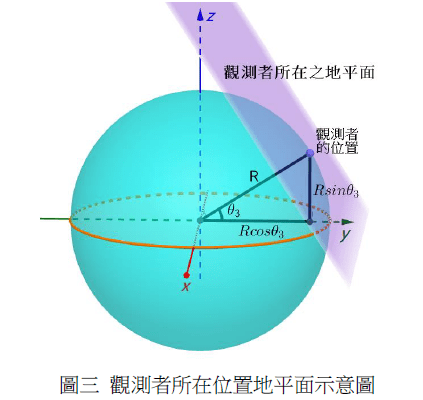(0，Rcosθ3，Rsinθ3)

(0，cosθ3，sinθ3)

(cosθ3)y+(sinθ3)z = R（地球半徑）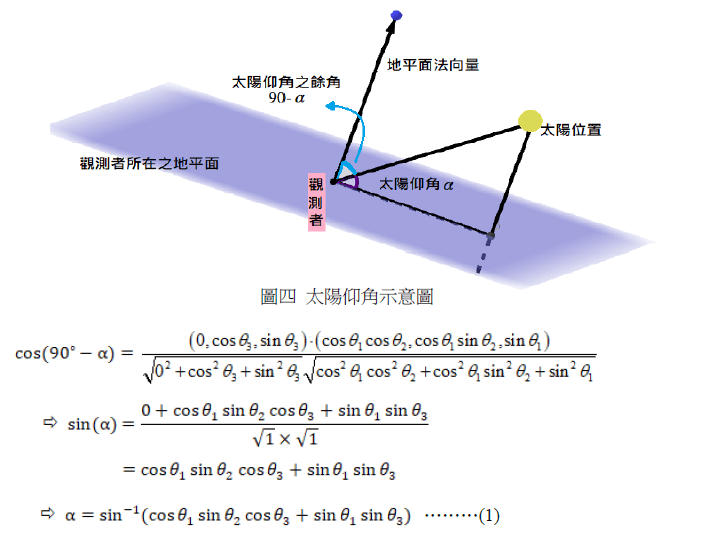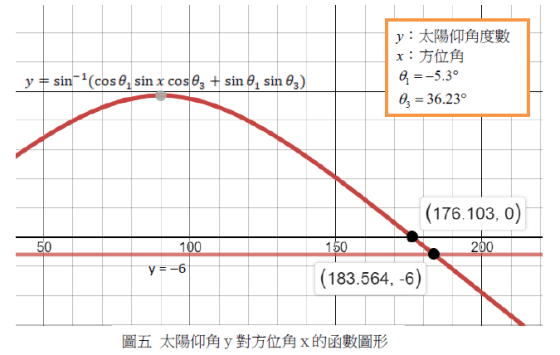(183.564-176.103)° = 7.461°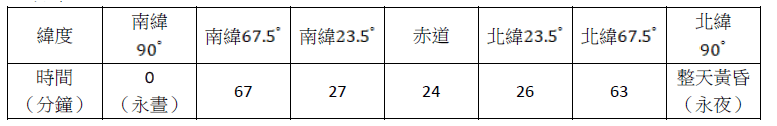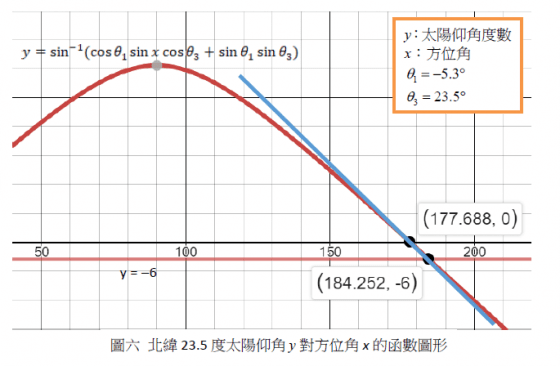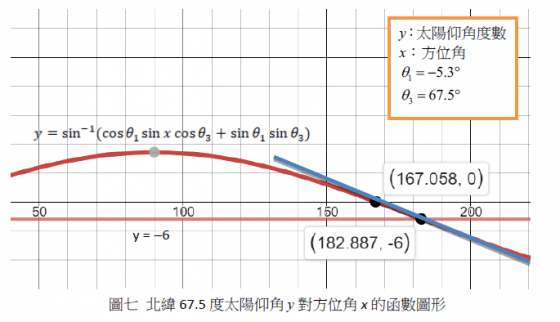\begin{equation}\left| m \right |=\left|\frac{y_2-y_1}{x_2-x_1} \right|=\left|\frac{**}{\Delta \theta_2} \right|\end{equation}

**為仰角變化量

## 四、結論與建議

「黃昏之時」有 29 分鐘，應足夠讓兩人寫完名字；但接下來會出現一個問題：瀧寫的不是名字，而是「我喜歡妳」。那麼，即使黃昏之時再久，三葉依舊無法得知瀧的名字。為什麼瀧要這麼做呢？

———————————————————————————————————————-

http://aa.usno.navy.mil/faq/docs/RST_defs.php

## 關於作者### PanSci

PanSci的編輯部帳號，會發自產內容跟各種消息喔。